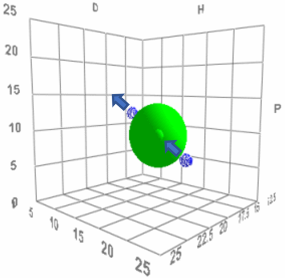# HSP and Temperature

HSP are based on cohesive energy density. If you raise the temperature then the density decreases so the HSP values go down. The effect depends on ΔT, the change of temperature from 25°C, and on the thermal expansion coefficient, α, which for simplicity in this app is set to 0.0007/K. The formulae (from Hansen and Beerbower, 1971) are:

δDT = δD*(1-ΔT*α*1.25)

δPT = δP*(1-ΔT*α/2)

δHT = δH*(1-ΔT*(0.00122+α/2))

Because of the difference in behaviour between small molecules and polymers, the app calculates two sets of values. First, it calculates the HSP of both molecules (1 and 2) at your chosen temperature as if they were small. Then it calculates two Distances. The first is if molecule 2 is a polymer keeping its HSP constant. The second is if molecule 2 is small. The differences in the distances are rather complex, depending on relative parameters. In general, small-small distances should change less because both HSP are changing. But because the different parameters change at different speeds, sometimes the small-small distance can change more than the small-polymer distance.

## Temperature Effects

δD1
δP1
δH1
T °C
δD1:δP1:δH1 @T
δD2:δP2:δH2 @T Small
δD2
δP2
δH2

Small-Polymer Distance
Small-Small DistanceThe small-polymer distance effect is interesting because there are two effects of temperature on the solubility of most polymers. The first is the general effect that for entropic reasons, higher temperatures give higher solubility. But the HSP-T effect can, in some cases, either increase or decrease solubility. Imagine the examples shown in the image where we have two bad solvents outside the Sphere. For both cases we can imagine that the arrows are pointing in the direction of lower HSP values. For the bad solvent which starts with high HSP values, increasing temperature takes the solvent into the Sphere and the polymer becomes soluble. But for the other solvent, increasing temperature takes the solvent further away from the Sphere. If (not shown) that solvent had started just inside the Sphere then increasing temperature will take it outside, so a "good" solvent for a polymer can become "bad" at higher temperatures. This effect is not very common but it has been regularly observed and puzzles many people who are unfamiliar with HSP!

The official site of Hansen Solubility Parameters and HSPiP software.